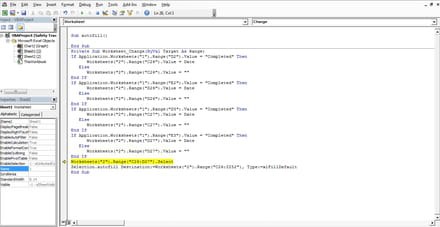# Macro auto fill is not working

Closed
Ashkan1984 Posts 1 Registration date Saturday May 14, 2016 Status Member Last seen May 14, 2016 - May 14, 2016 at 12:49 AM
Hi all
I am trying to write for my excel .I am begginer and this first time I tred Macro.
I wrote this code`Private Sub Worksheet_Change(ByVal Target As Range)If Application.Worksheets("1").Range("D2").Value = "Completed" Then      Worksheets("2").Range("C26").Value = Date   Else      Worksheets("2").Range("C26").Value = ""End IfIf Application.Worksheets("1").Range("E2").Value = "Completed" Then      Worksheets("2").Range("D26").Value = Date   Else      Worksheets("2").Range("D26").Value = ""End IfIf Application.Worksheets("1").Range("D3").Value = "Completed" Then      Worksheets("2").Range("C27").Value = Date   Else      Worksheets("2").Range("C27").Value = ""End IfIf Application.Worksheets("1").Range("E3").Value = "Completed" Then      Worksheets("2").Range("D27").Value = Date   Else      Worksheets("2").Range("D27").Value = ""End IfWorksheets("2").Range("C26:D27").SelectSelection.autofill Destination:=Worksheets("2").Range("C26:ZZ52")End Sub`

And i get this error:Run time error 1004;Select method of rang class failed and the line
Worksheets("2").Range("C26:D27").Select
is highlighted

I have no idea what to do ;can you help me thanks
Related: